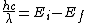# excitation potential

## Excitation potential

The difference in potential between an excited atomic or molecular state and the ground state. The term is most generally used in connection with electron excitation, but it can be applied to excited molecular vibrational and rotational states.

A closely related term is excitation energy. If the unit of potential is taken as the volt and the unit of energy as the electron volt, then the two are numerically equal. According to the Bohr theory, there is a relationship between the wavelength of the photon associated with the transition and the excitation energies of the two states. Thus the basic equation for the emission or absorption of energy is as shown below,where h is Planck's constant, c the velocity of light, λ the wavelength of the photon, and Ef and Ei the energies of the final and initial states, respectively. See Excited state, Ground state, Ionization potential

McGraw-Hill Concise Encyclopedia of Physics. © 2002 by The McGraw-Hill Companies, Inc.

## excitation potential

[‚ek‚sī′tā·shən pə‚ten·chəl]
(quantum mechanics)
Electric potential which gives the excitation energy when multiplied by the magnitude of the electron charge.
McGraw-Hill Dictionary of Scientific & Technical Terms, 6E, Copyright © 2003 by The McGraw-Hill Companies, Inc.
Site: Follow: Share:
Open / Close